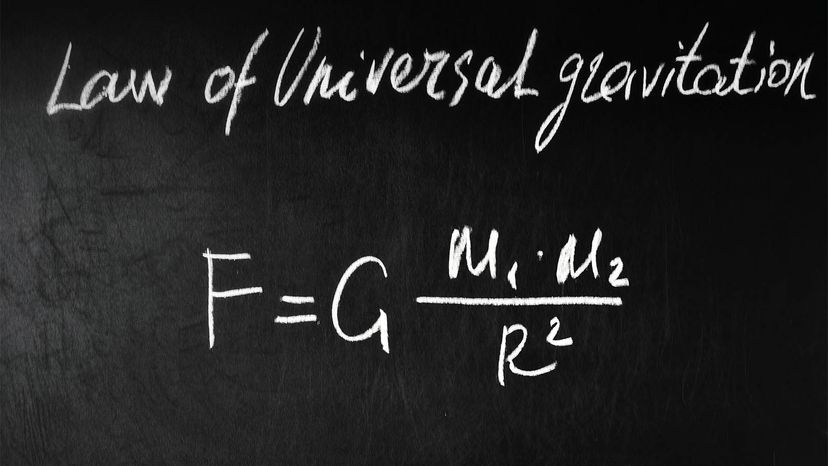# Gravitational Constant Is the "G" in Newton's Law of Universal Gravitation

By: Mark Mancini  |Isaac Newton proved that the force that causes an apple to fall to the ground is the same force that causes the moon to orbit the Earth. This is Newton's Law of Universal Gravitation, which he defined mathematically, using G as the gravitational constant. dmitro2009/Shutterstock

Here on the pale blue dot we call home, gravity is something we all experience every second of every day.

"Gravity is the glue that causes diffuse matter between the stars to slowly collapse and form new hydrogen-fusion machines (aka stars), it is the glue that binds galaxies together and it is responsible for our own Earth orbiting around the sun every year," says University of Connecticut astrophysicist Cara Battersby in an email.

Gravity was also the key player in Sir Isaac Newton's famous "apple" story You know the one we're talking about.

One day, Newton was hanging out in Lincolnshire, England when he watched an apple fall out of a tree. (Or so he claimed.)

Over the coming years, he'd tell many acquaintances — like Voltaire and biographer William Stukeley — that his great writings about the nature of gravity were inspired by this mundane little event. Newton's assistant John Conduitt, wrote:

[It] came into his thought that the same power of gravity (which made an apple fall from the tree to the ground) was not limited to a certain distance from the Earth but must extend much farther than was usually thought — Why not as high as the moon, said he to himself & if so that must influence her motion & perhaps retain her in her orbit.

Thus, the groundwork was laid for Newton's Law of Universal Gravitation. Central to which is a phenomenon called the gravitational constant, aka: "Big G" or just "G."

Contents

## The Equation

First thing's first. Before we tackle the Big G, we should step back and explain Newton's Law of Universal Gravitation.

As Katie Mack — an astrophysicist and the author of "The End of Everything (Astrophysically Speaking)" — says via email, gravity is "the mechanism by which things that have mass are attracted to each other."

Newton realized the strength of this attraction between a given set of objects depends on (a) how massive they are and (b) how far apart they are. It's a dynamic his Law of Universal Gravitation puts into mathematical terms.

Here's the relevant equation:

F = (G x m1 x m2) / r2

The "F" stands for the "force of gravity"; "m1" means the mass of the first object; "m2" denotes the mass of the second object; and "r2" is shorthand for the distance squared between the centers of mass within object one and object two.

And the "G"? Well friends, that's the Big G: The gravitational constant.

## "Golly, G!"

"For any two masses, be they bowling balls or planets, the gravitational force between them is determined by their masses, their distance and the number G," says Mack.

Thanks to experiments conducted by Henry Cavendish in the 1790s, we now know the gravitational constant has the numerical value of around 6.67 x 10 -11 Newtons (m2/kg2).

In this context, the term "Newtons" refers to a unit of measurement. One Newton is the amount of force you'll need to accelerate something with the mass of 2.2 pounds (1 kilogram) at 3.28 feet (1 meter) per second.

Like Anders Celsius and Charles F. Richter, Sir Isaac Newton earned a place on the vaunted list of scientists who've had units named in their honor. Good for him.Cygnus X-1 is a black hole about 15 times the mass of the sun in orbit with a massive blue companion star. Newton's description of gravity doesn't work for extremely strong gravity or very fast motion. In other words for things like black holes. NASA/CXC/M.Weiss

## Newton vs. Einstein

Now, there's a layer of nuance we should acknowledge here. You see, the Law of Universal Gravitation isn't quite as "universal" as the name implies.

According to Battersby, "our classic picture of gravity" — which Newton articulated back in the 17th century — is "an accurate approximation of the reality of physics in most places in the entire universe (certainly on Earth)."

"However," she adds, "this theory has been superseded by Einstein's Theory of General Relativity, which is an improvement on 'Newtonian Gravity,' that posits that matter deforms space-time itself (like a heavy ball creating a dimple on a rubber sheet)."

That brings us to black holes.

Capable of getting more than a million times more massive than our sun, black holes affect gravity in ways Newton's law just can't explain. General Relativity has been shown to make more accurate predictions about them.

"You have to start making corrections for the fact that Newton's description of gravity doesn't precisely work for extremely strong gravity or very fast motion," Mack says. "In those cases, we need to switch to Einstein's picture of gravity... But as long as you're not looking at one of those extreme cases, the equation that Isaac Newton wrote down in 1686 for what he called 'the Law of Universal Gravitation' truly is universal."